# Thermodynamics 2

12. Sep 2021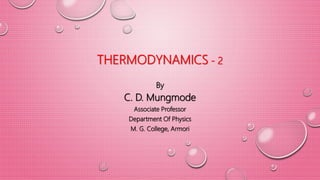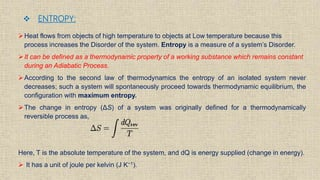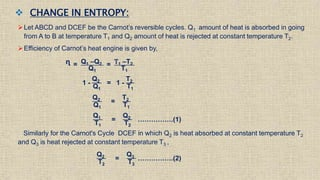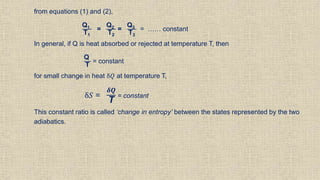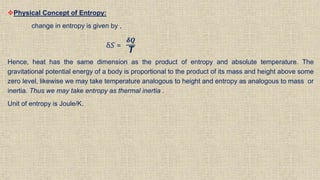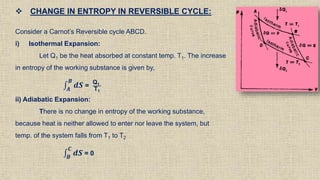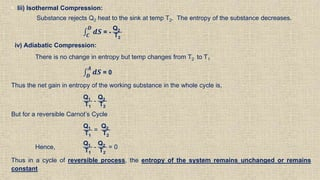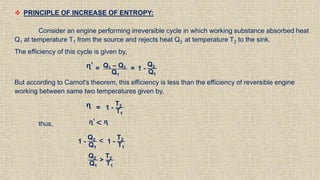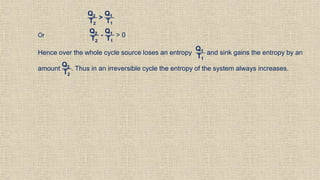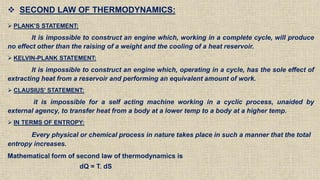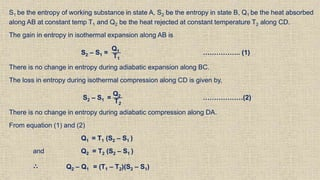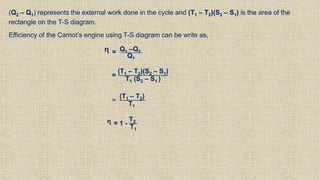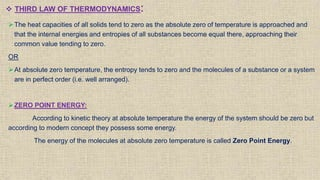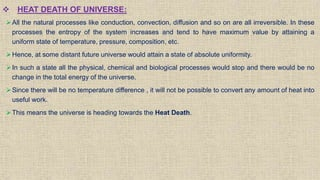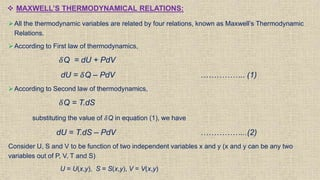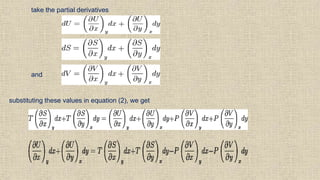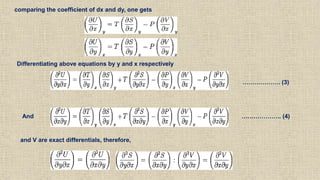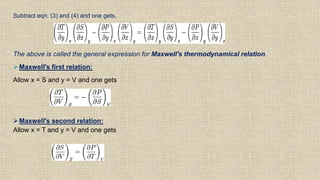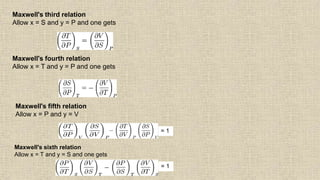1 von 20

### Thermodynamics 2

• 1. THERMODYNAMICS - 2 By C. D. Mungmode Associate Professor Department Of Physics M. G. College, Armori
• 2.  ENTROPY: Heat flows from objects of high temperature to objects at Low temperature because this process increases the Disorder of the system. Entropy is a measure of a system’s Disorder. It can be defined as a thermodynamic property of a working substance which remains constant during an Adiabatic Process. According to the second law of thermodynamics the entropy of an isolated system never decreases; such a system will spontaneously proceed towards thermodynamic equilibrium, the configuration with maximum entropy. The change in entropy (ΔS) of a system was originally defined for a thermodynamically reversible process as, Here, T is the absolute temperature of the system, and dQ is energy supplied (change in energy).  It has a unit of joule per kelvin (J K−1).
• 3.  CHANGE IN ENTROPY: Let ABCD and DCEF be the Carnot’s reversible cycles. Q1 amount of heat is absorbed in going from A to B at temperature T1 and Q2 amount of heat is rejected at constant temperature T2. Efficiency of Carnot’s heat engine is given by, ᶯ = Q1 –Q2 Q1 = T1 –T2 T1 1 - Q2 Q1 = 1 - T2 T1 Q2 Q1 = T2 T1 Q1 T1 = Q2 T2 …………….(1) Similarly for the Carnot's Cycle DCEF in which Q2 is heat absorbed at constant temperature T2 and Q3 is heat rejected at constant temperature T3 , Q2 T2 = Q3 T3 …………….(2)
• 4. from equations (1) and (2), Q1 T1 = Q2 T2 = Q3 T3 = …… constant In general, if Q is heat absorbed or rejected at temperature T, then Q T = constant for small change in heat δ𝑄 at temperature T, δ𝑆 = 𝜹𝑸 T = constant This constant ratio is called ‘change in entropy’ between the states represented by the two adiabatics.
• 5. Physical Concept of Entropy: change in entropy is given by , δ𝑆 = 𝜹𝑸 T Hence, heat has the same dimension as the product of entropy and absolute temperature. The gravitational potential energy of a body is proportional to the product of its mass and height above some zero level, likewise we may take temperature analogous to height and entropy as analogous to mass or inertia. Thus we may take entropy as thermal inertia . Unit of entropy is Joule/K.
• 6.  CHANGE IN ENTROPY IN REVERSIBLE CYCLE: Consider a Carnot’s Reversible cycle ABCD. i) Isothermal Expansion: Let Q1 be the heat absorbed at constant temp. T1. The increase in entropy of the working substance is given by, 𝑨 𝑩 𝒅𝑺 = Q1 T1 ii) Adiabatic Expansion: There is no change in entropy of the working substance, because heat is neither allowed to enter nor leave the system, but temp. of the system falls from T1 to T2 𝑩 𝑪 𝒅𝑺 = 0
• 7. • Iii) Isothermal Compression: Substance rejects Q2 heat to the sink at temp T2. The entropy of the substance decreases. 𝑪 𝑫 𝒅𝑺 = - Q2 T2 iv) Adiabatic Compression: There is no change in entropy but temp changes from T2 to T1 𝑫 𝑨 𝒅𝑺 = 0 Thus the net gain in entropy of the working substance in the whole cycle is, Q1 T1 - Q2 T2 But for a reversible Carnot’s Cycle Q1 T1 = Q2 T2 Hence, Q1 T1 - Q2 T2 = 0 Thus in a cycle of reversible process, the entropy of the system remains unchanged or remains constant
• 8.  PRINCIPLE OF INCREASE OF ENTROPY: Consider an engine performing irreversible cycle in which working substance absorbed heat Q1 at temperature T1 from the source and rejects heat Q2 at temperature T2 to the sink. The efficiency of this cycle is given by, ᶯ’ = Q1 – Q2 Q1 = 1 - Q2 Q1 But according to Carnot's theorem, this efficiency is less than the efficiency of reversible engine working between same two temperatures given by, ᶯ = 1 - T2 T1 thus, ᶯ’ < ᶯ 1 - Q2 Q1 < 1 - T2 T1 Q2 Q1 > T2 T1
• 9. Q2 T2 > Q1 T1 Or Q2 T2 - Q1 T1 > 0 Hence over the whole cycle source loses an entropy Q1 T1 and sink gains the entropy by an amount Q2 T2 . Thus in an irreversible cycle the entropy of the system always increases.
• 10.  SECOND LAW OF THERMODYNAMICS:  PLANK’S STATEMENT: It is impossible to construct an engine which, working in a complete cycle, will produce no effect other than the raising of a weight and the cooling of a heat reservoir.  KELVIN-PLANK STATEMENT: It is impossible to construct an engine which, operating in a cycle, has the sole effect of extracting heat from a reservoir and performing an equivalent amount of work.  CLAUSIUS’ STATEMENT: it is impossible for a self acting machine working in a cyclic process, unaided by external agency, to transfer heat from a body at a lower temp to a body at a higher temp.  IN TERMS OF ENTROPY: Every physical or chemical process in nature takes place in such a manner that the total entropy increases. Mathematical form of second law of thermodynamics is dQ = T. dS
• 11. T – S DIAGRAM: Thermodynamic changes in the state of a substance can be represented by plotting entropy (S) along horizontal axis and temperature (T) along perpendicular axis. Such a diagram is called temperature entropy (T-S) diagram. Consider Carnot’s reversible cycle. On T-S diagram , isothermal curves are shown by two straight lines AB and CD parallel to S - axis. The adiabatic curves are shown by the straight lines BC and DA parallel to T - axis. Fig. A P-V diagram of the Carnot Cycle. Fig. A T-S diagram of the Carnot Cycle. A B C D S2
• 12. S1 be the entropy of working substance in state A, S2 be the entropy in state B, Q1 be the heat absorbed along AB at constant temp T1 and Q2 be the heat rejected at constant temperature T2 along CD. The gain in entropy in isothermal expansion along AB is S2 – S1 = Q1 T1 …………….. (1) There is no change in entropy during adiabatic expansion along BC. The loss in entropy during isothermal compression along CD is given by, S2 – S1 = Q2 T2 ………………(2) There is no change in entropy during adiabatic compression along DA. From equation (1) and (2) Q1 = T1 (S2 – S1 ) and Q2 = T2 (S2 – S1 ) ∴ Q2 – Q1 = (T1 – T2)(S2 – S1)
• 13. (Q2 – Q1) represents the external work done in the cycle and (T1 – T2)(S2 – S1) is the area of the rectangle on the T-S diagram. Efficiency of the Carnot’s engine using T-S diagram can be write as, ᶯ = Q1 –Q2 Q1 = (T1 – T2)(S2 – S1) T1 (S2 – S1 ) = (T1 – T2) T1 ᶯ = 1 - T2 T1
• 14.  THIRD LAW OF THERMODYNAMICS: The heat capacities of all solids tend to zero as the absolute zero of temperature is approached and that the internal energies and entropies of all substances become equal there, approaching their common value tending to zero. OR At absolute zero temperature, the entropy tends to zero and the molecules of a substance or a system are in perfect order (i.e. well arranged). ZERO POINT ENERGY: According to kinetic theory at absolute temperature the energy of the system should be zero but according to modern concept they possess some energy. The energy of the molecules at absolute zero temperature is called Zero Point Energy.
• 15.  HEAT DEATH OF UNIVERSE: All the natural processes like conduction, convection, diffusion and so on are all irreversible. In these processes the entropy of the system increases and tend to have maximum value by attaining a uniform state of temperature, pressure, composition, etc. Hence, at some distant future universe would attain a state of absolute uniformity. In such a state all the physical, chemical and biological processes would stop and there would be no change in the total energy of the universe. Since there will be no temperature difference , it will not be possible to convert any amount of heat into useful work. This means the universe is heading towards the Heat Death.
• 16.  MAXWELL’S THERMODYNAMICAL RELATIONS: All the thermodynamic variables are related by four relations, known as Maxwell’s Thermodynamic Relations. According to First law of thermodynamics, 𝛿Q = dU + PdV dU = 𝛿Q – PdV …………….. (1) According to Second law of thermodynamics, 𝛿Q = T.dS substituting the value of 𝛿Q in equation (1), we have dU = T.dS – PdV ……………...(2) Consider U, S and V to be function of two independent variables x and y (x and y can be any two variables out of P, V, T and S) U = U(x,y), S = S(x,y), V = V(x,y)
• 17. take the partial derivatives and substituting these values in equation (2), we get
• 18. comparing the coefficient of dx and dy, one gets Differentiating above equations by y and x respectively And ……………….. (4) and V are exact differentials, therefore, ………………. (3)
• 19. Subtract eqn. (3) and (4) and one gets, The above is called the general expression for Maxwell's thermodynamical relation. Maxwell's first relation: Allow x = S and y = V and one gets Maxwell's second relation: Allow x = T and y = V and one gets
• 20. Maxwell's third relation Allow x = S and y = P and one gets Maxwell's fourth relation Allow x = T and y = P and one gets Maxwell's fifth relation Allow x = P and y = V = 1 Maxwell's sixth relation Allow x = T and y = S and one gets = 1